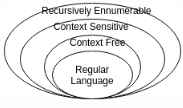Courses

# Test: Context Free Grammar Derivations And Definitions

## 10 Questions MCQ Test Theory of Computation | Test: Context Free Grammar Derivations And Definitions

Description
This mock test of Test: Context Free Grammar Derivations And Definitions for Computer Science Engineering (CSE) helps you for every Computer Science Engineering (CSE) entrance exam. This contains 10 Multiple Choice Questions for Computer Science Engineering (CSE) Test: Context Free Grammar Derivations And Definitions (mcq) to study with solutions a complete question bank. The solved questions answers in this Test: Context Free Grammar Derivations And Definitions quiz give you a good mix of easy questions and tough questions. Computer Science Engineering (CSE) students definitely take this Test: Context Free Grammar Derivations And Definitions exercise for a better result in the exam. You can find other Test: Context Free Grammar Derivations And Definitions extra questions, long questions & short questions for Computer Science Engineering (CSE) on EduRev as well by searching above.
QUESTION: 1

### The entity which generate Language is termed as:

Solution:

The entity which accepts a language is termed as Automata while the one which generates it is called Grammar. Tokens are the smallest individual unit of a program.

QUESTION: 2

### Production Rule: aAb->agb belongs to which of the following category?

Solution:

Context Sensitive Language or Type 1 or Linearly Bounded Non deterministic Language has the production rule where the production is context dependent i.e. aAb->agb.

QUESTION: 3

### Which of the following statement is false?

Solution:

Explanation: Every regular language can be produced by context free grammar and context free language can be produced by context sensitive grammar and so on.QUESTION: 4

The Grammar can be defined as: G=(V, ∑, p, S)In the given definition, what does S represents?

Solution:

G=(V, ∑, p, S), here V=Finite set of variables, ∑= set of terminals, p= finite productions, S= Starting Variable.

QUESTION: 5

Which among the following cannot be accepted by a regular grammar?

Solution:

There exists no finite automata to accept the given language i.e. 0n1n. For other options, it is possible to make a dfa or nfa representing the language set.

QUESTION: 6

Which of the expression is appropriate?For production p: a->b where a∈V and b∈_______

Solution:

According to the definition, the starting variable can produce another variable or any terminal or a variable which leads to terminal.

QUESTION: 7

For S->0S1|e for ∑={0,1}*, which of the following is wrong for the language produced?

Solution:

L={e, 01, 0011, 000111, ……0n1n }. As epsilon is a part of the set, thus all the options are correct implying none of them to be wrong.

QUESTION: 8

The minimum number of productions required to produce a language consisting of palindrome strings over ∑={a,b} is

Solution:

The grammar which produces a palindrome set can be written as:
S-> aSa | bSb | e | a | b
L={e, a, b, aba, abbbaabbba…..}

QUESTION: 9

Which of the following statement is correct?

Solution:

Regular grammar is a subset of context free grammar and thus all regular grammars are context free.

QUESTION: 10

Are ambiguous grammar context free?

Solution:

A context free grammar G is ambiguous if there is atleast one string in L(G) which has two or more distinct leftmost derivations.